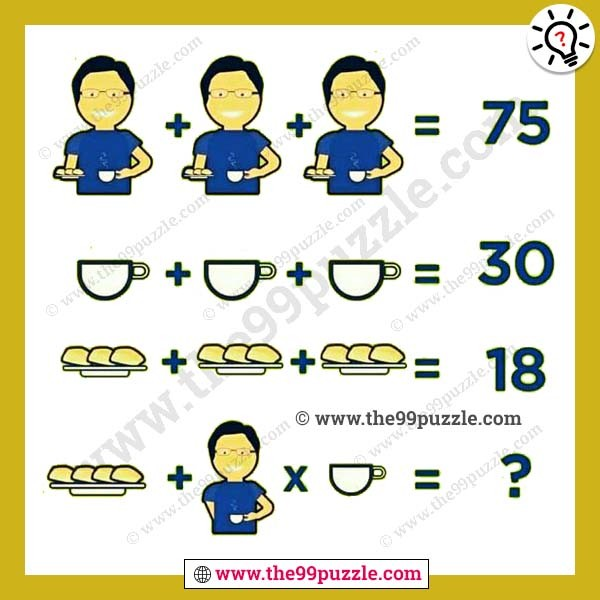# Man, biscuit, cup math picture equation – Puzz138

Man, biscuit, cup math picture equation for adults. This visual math puzzle can improve IQ knowledge and visual power. This brain teaser picture puzzle can challenge your mind. See the picture carefully and find out the last equation value of the Man, biscuit, cup math picture equation for adults.###### Explanation:

Man(without cup and biscuit)=9

Each biscuit=2

Each cup=10

25+25+25=75

10+10+10=30

(2×3)+(2×3)+(2×3)=18

(2×4)+(9+10)×10=198